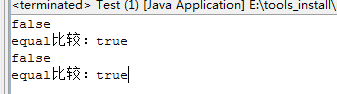# Java中==与equal的区别

#### Java中==与equal的区别

``````String str1 = new String("str");
String str2 = new String("str");
System.out.println("==比较 ："+ (str1 == str2));
System.out.println("equal比较："+ str1.equals(str2));
String str3 = "str1";
String str4 = "str1";
System.out.println("==比较 ："+ (str3 == str4));
System.out.println("equal比较："+ str3.equals(str4));
````````````false
equal比较：true
true
equal比较：true
``````

``````public boolean equals(Object obj) {
return (this == obj);
}
``````

``````public boolean equals(Object anObject) {
if (this == anObject) {
return true;
}
if (anObject instanceof String) {
String anotherString = (String)anObject;
int n = count;
if (n == anotherString.count) {
char v1[] = value;
char v2[] = anotherString.value;
int i = offset;
int j = anotherString.offset;
while (n-- != 0) {
if (v1[i++] != v2[j++])
return false;
}
return true;
}
}
return false;
}
``````

equal:是用来比较两个对象内部的内容是否相等的，由于所有的类都是继承自java.lang.Object类的，所以如果没有对该方法进行覆盖的话，调用的仍然是Object类中的方法，而Object中的equal方法返回的却是==的判断，因此，如果在没有进行该方法的覆盖后，调用该方法是没有任何意义的。

==：是用来判断两个对象的地址是否相同，即是否是指相同一个对象。比较的是真正意义上的指针操作。 转载原文 http://www.cnblogs.com/shenliang123/archive/2012/04/16/2452156.html

### 相关文章

##### 关注道招网公众帐号